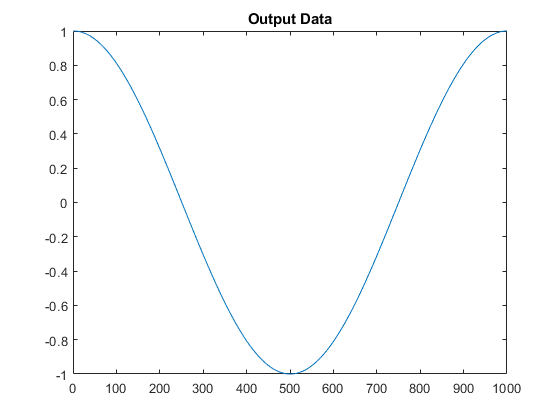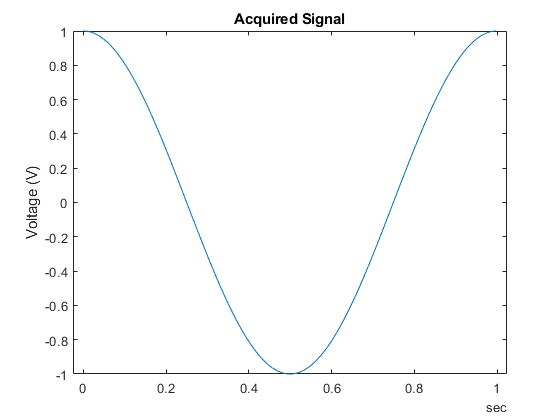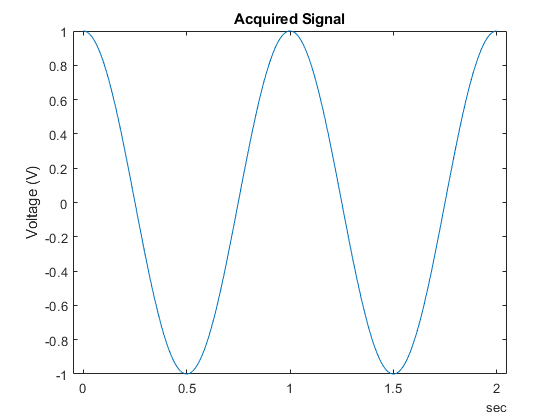Documentation

This is machine translation

Mouseover text to see original. Click the button below to return to the English version of the page.

Acquire Data and Generate Signals at the Same Time

This example shows how to use analog input channels and analog output channels together with a single Session object running near simultaneous.

Create a Session Object

Use `daq.createSession` to create a session.

```s = daq.createSession('ni') ```
```s = Data acquisition session using National Instruments hardware: Will run for 1 second (1000 scans) at 1000 scans/second. No channels have been added. ```

Setup Hardware

For the purpose of this example use a compactDAQ chassis NI c9172 with NI 9205 (cDAQ1Mod1 - 4 analog input channels) module and NI 9263 (CDAQ1Mod2 - 4 analog output channels) module. Use the `daq.getDevices` function to get more information about connected hardware. For more information see the Discover NI Devices Using the Session-Based Interface example.

In this example the analog output channels are connected back to the analog input channels so that the acquired data is same as the data generated from the analog output channel.

Use the `addAnalogInputChannel` command to add an analog input voltage channel to the session object for acquisition.

```addAnalogInputChannel(s,'cDAQ1Mod1',0,'Voltage') ```
```ans = 1×365 char array Data acquisition session using National Instruments hardware: Will run for 1 second (1000 scans) at 1000 scans/second. Number of channels: 1 index Type Device Channel MeasurementType Range Name ----- ---- --------- ------- --------------- ---------------- ---- 1 ai cDAQ1Mod1 ai0 Voltage (Diff) -10 to +10 Volts ```

Use the `addAnalogOutputChannel` command is used to add an analog output voltage channel to the session for sending data.

The Session objects can contain channels of different types in the same object and can be used to start acquisition and generate signals at the same time.

```addAnalogOutputChannel(s,'cDAQ1Mod2',0,'Voltage') ```
```ans = 1×424 char array Data acquisition session using National Instruments hardware: No data queued. Will run at 1000 scans/second. Number of channels: 2 index Type Device Channel MeasurementType Range Name ----- ---- --------- ------- --------------- ---------------- ---- 1 ai cDAQ1Mod1 ai0 Voltage (Diff) -10 to +10 Volts 2 ao cDAQ1Mod2 ao0 Voltage (Diff) -10 to +10 Volts ```

Queuing Output Data for the Analog Output Channel

The analog output channel you just added shows that no data is queued for the object. This is because analog output channels require data to be queued before the operation is started.

A sine wave signal is generated and queued. Note that for each analog output channel the data is in a separate column.

```output_data = sin(linspace(0,2*pi,1000)'); ```

Use the `queueOutputData` command to queue the data.

```queueOutputData(s,output_data) ```

Plot the output data.

```plot(output_data); title('Output Data Queued'); ```Start Acquisition and Plot Data

Once the data is queued, you can start operations in the foreground or background. Foreground operations block MATLAB® until the operations are complete and data is returned. Background operations are non blocking and return control to MATLAB command line processing immediately, allowing other MATLAB code to run in parallel.

Use the `startForeground` command to start operations in the foreground. Both generation of signal (analog output) and acquiring of signal (analog input) are synchronized and start together. You can see this from the acquired data being the same as the generated data as the analog output channels are connected back to the analog input channels.

```[captured_data,time] = s.startForeground(); ```

Plot the acquired data acquired and see that it is exactly the same as generated data.

```plot(time,captured_data); ylabel('Voltage'); xlabel('Time'); title('Acquired Signal'); ```Change the Length of Operation

The duration of acquisition depends upon the amount of data queued for the analog output channel. Since both the channels run at the same rate of 1000 scans per second, the duration of operation, after queuing 1000 data points above is 1 second as shown `DurationInSeconds`.

```output_data = 2*sin(linspace(0,2*pi,1000)'); % Amplitude of 2V queueOutputData(s,output_data); duration = s.DurationInSeconds ```
```duration = 1 ```

If you queue more data, the duration of operation will change automatically.

```output_data = 2*sin(linspace(2*pi,3*pi,500)'); queueOutputData(s,output_data); duration = s.DurationInSeconds ```
```duration = 1.5000 ```

Use the `startForeground` command to start the acquisition and the generation simultaneously.

```[captured_data,time] = s.startForeground(); ```

Plot the acquired data acquired. It is exactly the same as generated data.

```plot(time,captured_data); ylabel('Voltage'); xlabel('Time'); title('Acquired Signal'); ```Data Acquisition Toolbox DocumentationGet trial now